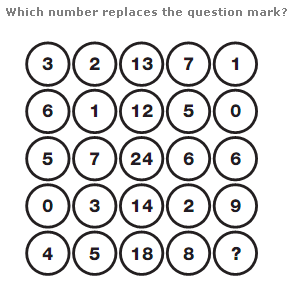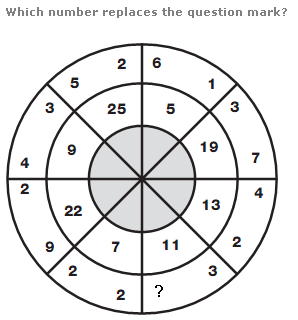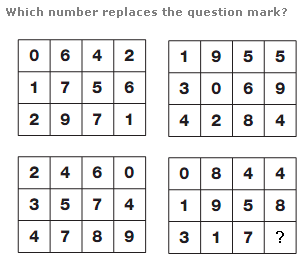# Puzzles - Number puzzles

### Exercise :: Number puzzlesAnswer : 1 Explanation : Working through the diagram in rows, the central value equals the sum of the other numbers in the row.Answer : 8 Explanation : Multiply the 2 numbers in the outer segments together and add 1 to give the value in the inner segment opposite.Answer : 3 Explanation : Split each grid into 2 halves, vertically, leaving 2 columns, each 2 squares wide. Start with the top left pair and move down, then to the top right pair, and down again. Reading each pair of digits as 2 digit values, the 2 digit values increase by 11, 12, 13, 14 and 15.